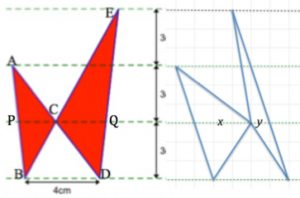Find the total area of the two triangles by two different methods if ACD and BCE are straight lines .

What are the lengths PC and CQ?

The triangles are re-drawn keeping the total area the same and keeping the distances between the green parallel lines as 3 cm and BD = 4 cm.What is the value of y if x = 3 cm?

What other values can x and y take if the area is always the same?

This activity is adapted from the NRICH task Kissing Triangles with permission of the University of Cambridge. All rights reserved.

Tagged with: